Smartick is an advanced online program that teaches kids math and coding in only 15 min. a day

Oct27

# Understanding Division with the Help of Geometric Visualization

We’ve written plenty of posts about division on the Smartick blog. The division algorithm, sometimes, can be difficult or a pretty long-winded way of calculating, especially if the divisor contains 2 or more digits.

### Understanding division with the help of geometric visualization

In this post, we’re going to view division as the opposite operation to multiplication, and we’re going to use blocks to help us visualize it.

To start with, we need to know what each of the blocks we’re going to use represents. The little square represents a unit, the bar represents a ten, and the big square or flat represents a hundred.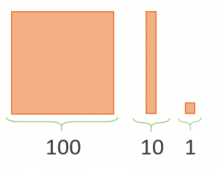Now, before dividing, we’re going to learn how to multiply with the blocks. For example, we’re going to multiply 21 by 13. To do this, first we have to represent each number with the blocks: the 21 is made up of two tens and a unit, and the 13 is made up of one ten and three units. We arrange them in the following way: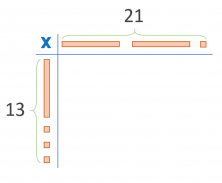Now we draw the results of the multiplication. By multiplying 2 tens we get a hundred, as the next image shows.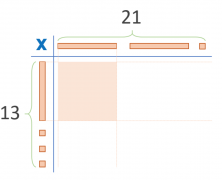We get the following image by repeating the process for all the blocks.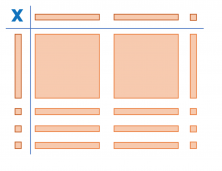Using this image, we can say that the result of 21 x 13 is equal to 273 (2 hundreds, 7 tens, and 3 units).

## 264 ÷ 12

We start by placing the number 12 in the multiplication table. On the other side, we draw the blocks that make up the number 264.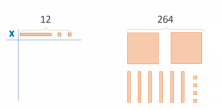We have to put the blocks on the right into the table on the left, in the correct positions. We start with the hundreds. The only possible option we have is to put them under the ten of the number 12.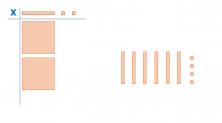Now we place the tens.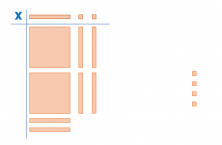And finally, we place the units.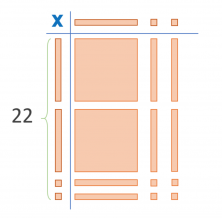Perfect! We’ve ended up with the number 22. This means that 264 ÷ 12 = 22.

Have you found this way of dividing interesting? Try with other numbers and see if you get the right answer. You’ll see how fun it can be!

If you want to keep learning math, register with Smartick and try it free!